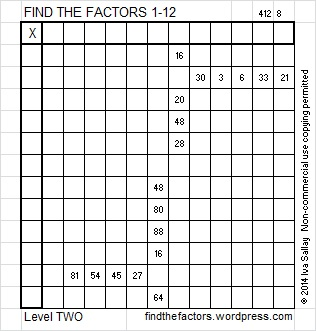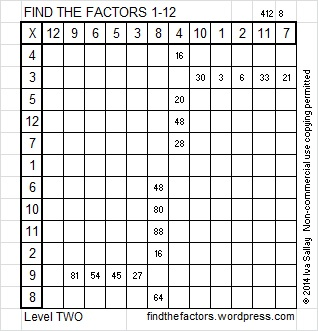# 70 and Level 2This week’s puzzles for you to solve

70 is a composite number. 70 = 1 x 70, 2 x 35, 5 x 14, and 7 x 10. Factors of 70: 1, 2, 5, 7, 10, 14, 35, 70. Prime factorization: 70 = 2 x 5 x 7.Sometimes 70 is a clue in the FIND THE FACTORS 1 – 12 puzzles. Even though it has other factors, we only use 70 = 7 x 10 in the puzzles.

Here are the factors for today’s puzzle: